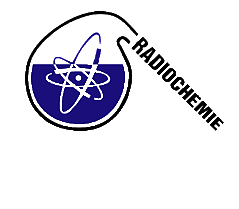#RadChem 2014

May 11 – 16, 2014
Casino Conference Centre
Europe/Prague timezone

## Theoretical Chemistry Study of Uranyl (VI)-Sulphate complex species

May 13, 2014, 5:15 PM
1h 30m
Casino Conference Centre

#### Casino Conference Centre

Reitenbergerova 4/95, Mari&#225;nsk&#233; L&#225;zn&#283;, Czech Republic <font color=white>
Poster Chemistry of Actinide and Trans-actinide Elements

### Speaker

Mr Jakub Visnak (Department of Nuclear Chemistry, Faculty of Nuclear Sciences and Physical Engineering, Czech Technical University in Prague)

### Description

This theoretical study of several possible complex species of the general formula ([UO$_2$(H$_2$O)$_a$($\eta^1$-SO$_4$)$_b$($\eta^2$-SO$_4$)$_c$]$^{2-2(b+c)}, 0 ≤ b + c ≤ 4, 5 ≤ a + b + 2c ≤ 6, [UO$_2$(H$_2$O)$_2$($\eta^1$-SO$_4$)($\eta^2$-SO$_4$)]$^{2-}$depicted at the Fig. 1.) aspires to the theoretical prediction of stability constants$\beta$°, thermodynamical properties (i.e.$\Delta$G° and$\Delta$H°) and their dependance on the temperature. Equilibrium geometries in the ground state and several excited electronic states, vibrational modes and frequencies, moments of inertia, vertical transition energies important to the UV-VIS absorption, excitation and emission/fluorescence spectra were calculated as well. Our study is also the first step towards the molecular-dynamical study searching seeking for another approach to predict$\Delta$G°,$\Delta$H° and log$\beta\$° and, more importantly, to predict the fluorescence lifetimes (or more generaly - the TRLFS spectra) and their dependance on the temperature and ionic strength (or, more generaly - the solution composition). The comparison of all of the ab initio predicted and experimentally obtained quantities is included. Both, the purely ab initio methods based on the Dirac(-Breit) equation (DHF, MCDHF, KRCI, CCSD) and the density functional methods (DFT (b3-lyp/def-SVP), TDDFT (bh-lyp/def-SVP)) were used. This work tries to reproduce and further develop the study of uranyl-sulfates done by Jakub Šebera .

### Primary authors

Mr Jakub Visnak (Department of Nuclear Chemistry, Faculty of Nuclear Sciences and Physical Engineering, Czech Technical University in Prague) Mr Lukas Sobek (Department of Nuclear Chemistry, Faculty of Nuclear Sciences and Physical Engineering, Czech Technical University in Prague)

### Presentation materials

There are no materials yet.